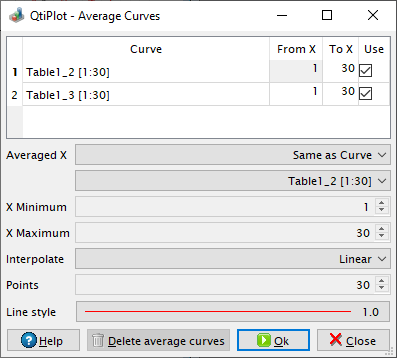# Average Multiple Curves Dialog

This dialog is activated by selecting the Average Multiple Curves... command from the Analysis menu.

The table at the top of the dialog displays all the curves available in the active plot layer and their X data range. It allows users to select the curves to be averaged, by checking the corresponding box from the Use column.

Figure 5-105. The Average Multiple Curves dialog.The Averaged X list box can be used in order to specify how X values will be computed for the averaged output curve. There are four methods available:

1. Same as Curve: uses the X values of the data curve selected in the nearby list box. For the selected curve, the exact Y values are used when calculating the average. For all other datasets the Y values are interpolated.

2. Common X Range: generates a set of equally spaced X values from the largest minimum X to the smallest maximum X and calculates the average of all interpolated Y.

3. Full X Range: generates a set of equally spaced X values from the smallest minimum X to the largest maximum X and calculates the average of all interpolated Y.

4. Custom: generates a set of equally spaced values within the specified X range and calculates the average of all interpolated Y.

The X Minimun box displays the lower limit of the X range for the averaged curve. It can be edited only when the method selected in the Averaged X list box is Custom, otherwise it is read-only.

The X Maximum box displays the upper limit of the X range for the averaged curve. It can be edited only when the method selected in the Averaged X list box is Custom, otherwise it is read-only.

The Interpolate list box can be used to control the interpolation method. Three methods are available: Linear, Cubic and Non-rounded Akima. Please note that each of these methods require a minimum number of data points in the input curves which is 2, 3 and 5 respectively.

The Points box is read-only if the method selected in the Averaged X list box is Same as Curve. Otherwise it can be used to specify the number of points for the interpolated curves.

The Color box can be used to specify the color of the output curve.

If the Delete average curves button is pressed all output curves generated during the current session of the dialog are deleted.